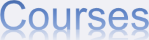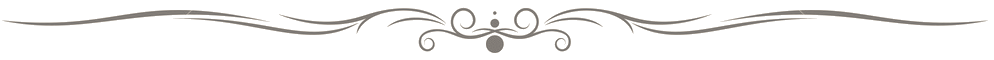﻿

1) Overview (1000-level math courses)

Math Department currently offers five sequences of single variable calculus courses at different levels and with different intensions. They are:

 MATH1012*/1014 MATH1013*/1014 MATH1023*/1024 MATH1020* (M2 with 5* or above) MATH1003*

The first three are year long sequences, and the last two are semester long courses.
* common core courses under QR

2) Purpose of each course

MATH1012-1014: Complete single variable calculus for students without M1 and M2. Students are expected to know the basic concepts and carry out the computations.

MATH1013-1014: Complete single variable calculus for students with M1/M2, or equivalent background. Students are expected to know the basic concepts and carry out the computations.

MATH1023-1024: Complete single variable calculus at more advanced level. Students are expected to understand the basic concepts and make simple theoretical arguments (such as providing reason for the specific computation, quite a bit of proofs). This is an honor course version of Math1013/1014.

(Student with a level 5 or above in HKDSE Mathematics Extended Module M2 are encouraged to try out these honor classes)

MATH1020: Advance material in single variable calculus. Expected students have learnt well in calculus before (M2 with 5* or above). The course contains advance material so that students who passed Math1020 can continue studying the other higher level math courses.

MATH1003: Minimum material in single variable calculus. The course provides the basic knowledge of calculus but not enough for further study in more advanced math courses.

3) Relation between different courses

MATH1023-1024 >MATH1013/1014  = MATH1020 > MATH1012-MATH1014 > MATH1003

If you pass MATH1023 in fall, then you can take either MATH1024 or MATH1014 in spring.

If you pass MATH1012 or MATH1013 in fall, then you can take MATH1014 in spring. If you perform exceptionally well in MATH1013, then you can take MATH1024 in spring.

If you pass MATH1020 or MATH1003, then you cannot take other single variable calculus courses, except that students with grade A- or above in MATH1003 may take MATH1014.

4) How does the choice affect further study

MATH(1012/1013) / 1014 / (1023/1024) / 1020 is the prerequisite for

MATH2043 (honor analysis course) or

MATH2031/2033 (the normal analysis course).

Although according to the official rule, getting A- or above in

MATH1014 / 1020 / 1024 can allow you to register MATH2043,

it is strongly advised that only those who took MATH1023/1024

(or MATH1020) continue with MATH2043

because of the level of difficulty of MATH2043. The same applies to MATH2131 (another honor course).

Students intend to do research in Math are strongly advised to take MATH1023/MATH1024.

5) Other math courses that are offered at different levels

The following is the list of other math courses that are offered at different levels.

MATH2023 > MATH2011
MATH2131 > MATH2121
MATH2352 > MATH2351
MATH2043 > MATH2033

For further enquiry, contact Dr. Tsz-Kin Lam at tklam@ust.hk